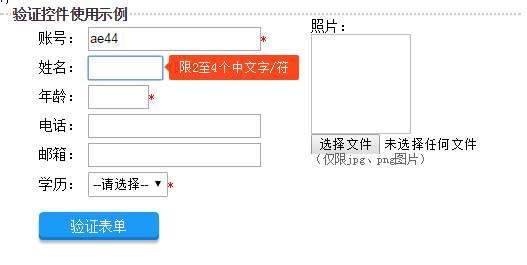# Js表单验证控件（使用方便，无需编码）-01使用说明

1、支持的验证：

2、其它功能：

示例：<form onsubmit="alert('验证成功');return false;">
<p>姓名：<input name="" type="text" size="6" datatype="chinese" lenlimit="2-4" alt="限2至4个中文字/符"><span>（限中文）</span>
</p>
<p>  <input type="submit" name="button" class="button" value="验证表单" verify="true"/>  </p>
</form>


1、非空验证

<input name="" type="text" nullable="false"/>

2、字符长度验证

<input name="" type="text" lenlimit="5"/>（最长5个字符）

<input name="" type="text" lenlimit="5-10"/>（最少5个字符，最多10个字符）

3、数值区间验证

<input name="" type="text" numlimit="5"/>（数值不得大于5）

<input name="" type="text" numlimit="5-10"/>（数值介于5-10之间）

指定某个录入控件为设定值

<p> 产品价格：
<input name="prise" type="text" value="10" datatype="uint"/>
元 </p>
<p>最低优惠：
<input name="min" type="text" value="12"
datatype="uint" numlimit="{prise}"/>(优惠价不可以超过产品价格)
</p>
<p> 最大优惠：
<input name="max" type="text"
numlimit="{min}-{prise}"/>(最大优惠大于最低优惠，但不可以超过产品价格)
</p>


4、输入是否相同

<input name="pw1" type="text"/>

<input name="pw2" type="text" sametarget="pw1"/>（与name="pw1"的控件录入相同）

5、不等于某值

<select name="select" id="select" novalue="|1|2" alt="请选择专科或以上学历">        <option value="">--请选择--</option>        <option value="5">硕士</option>        <option value="4">本科</option>        <option value="3">专科</option>        <option value="2">中学</option>        <option value="1">小学</option>      </select>

6、数据类型验证

<input name="tm" type="text" datatype="uint"/> 设置datatype="uint"，要求录入正整数。

7、文件类型限制

<input name="" type="file" fileallow="jpg|png"/>，只允许上传jpg与png图片

<input name="" type="file" filelimit="exe|com|bat|js"/>，禁止上传可执行文件

8、首尾字符验证

<input name="" type="text" begin="http|https"/>（必须是网址）

<input name="" type="text" end="@qq.com"/>（必须是qq邮箱）

9、正则表达式验证

<input name="" type="text" regex="\d+"/>（全部为数字）

10、分组验证

<input type="text" datatype="email" nullable="false" group="a"/>

<input type="submit" name="button" class="button" value="验证表单" verify="true" group="a" />

<p>电话：
<input type="text" datatype="mobile|tel" nullable="false"  group="a|c">（同时属于a组和c组）
</p>
<p>

</p>
<input type="submit" name="button" class="button" value="验证A组和B组" verify="true" group="a|b"/>
<input type="submit" name="button" class="button" value="验证B组和C组" verify="true" group="b|c"/>


11、验证方式

12、提示信息样式设置

nullable false或true true 是否允许为空，默认是允许，即为true。当为false时，控件右侧显会显示红色星号。（如果设置star='false'则不显示）
star false或true true 是否允许显示红色星号，为false时不显示。
lenlimit

lenlimit="5"

lenlimit="5-10"

lenlimit="5"，录入字符最长不得超过5个字符，包括5个

lenlimit="5-10"，录入字符长度为5至10个字符，包括5与10

numlimit

numlimit="5"

numlimit="5-10"

numlimit="｛ctlname｝"

numlimit="5"，录入数值最大不得超过5，包括5个

numlimit="5-10"，录入5至10之间的数值，包括5与10

numlimit="｛ctlname｝"，录入数值不超过控件名称为ctlname的值

sametarget 要比较的控件的name值   最常见的应用场景就是密码输入，此处填写要比较录入值的控件的name
novalue

要求控件的值不得等于某些值，如novalue="1|2"，即不得等于1和2。
datatype chinese 中文
user 账户
uint 正整数
number 数字
float 浮点数
tel 电话
mobile 移动电话
zip 邮编
email 电子邮箱
idcard 身份证
qq 号
url 网址
验证录入的数据是否符合要求的数据类型。

fileallow

允许上传的文件类型。最常见的应用场景是文件上传
filelimit

禁止上传的文件类型。最常见的应用场景是文件上传
begin

限定必须以某值开头，常见的应用场景，如录入网址，可以http://开头或https://开头。如begin="http|https"
end

限定必须以某值结尾，end="@qq.com|@163.com"，必须是qq邮箱或163邮箱
group

all验证所有

patter sumbit或focus sumbit

place top、bottom、left、right bottom

bgcolor 颜色值 红色

fgcolor 颜色值 白色

posted @ 2018-03-13 10:50  songsoft  阅读(1994)  评论(2编辑  收藏  举报